# Outlier Detection via Markov Chain Monte Carlo

Previously, I wrote outlier detection using FFT and Median Filtering and this post will be second in that series where I will look at the outlier detection in time-series using Markov Chaing Monte Carlo(MCMC). If you are familiar with Python and want to use MCMC, you should definitely check out PyMC, which I generally use for Bayesian Modeling and MCMC in Python. Although my main focus is the outliers, since I will be using a linear signal as an example, this method could be also used as a robust linear regression to ignore the outliers and focus on the main signal.

I will give a talk next week in PyData(on Sunday) focusing on these approaches for outlier detection in time series signals, use go2-PYDATA code to get 15% off if you are not yet registered.

After shameless promotion, let's get started.

In :
import numpy as np
import scipy
import pymc
import random
from matplotlib import pyplot as plt

Couldn't import dot_parser, loading of dot files will not be possible.

In :
a = 2
x = np.arange(10,50,.5)
x += np.random.normal(size=x.size)
y = a * x + 10

#y = a * np.sin(x) + 5
y_with_outlier = np.copy(y)

for ii in np.arange(len(x)/5, len(x), len(x)/5.):
y_with_outlier[ii]= 60*(random.random()-.5) + y[ii]

y_with_outlier = np.asarray(y_with_outlier)
# assuming I do not know, just fill it with ones
spread = np.asarray([1 for _ in range(y_with_outlier.size)])


I put some random noise to the signal before introducing the outliers as I want to preserve the small variance but not very distant ones.

In :
plt.figure(figsize=(12, 6));
plt.scatter(range(len(y)), y, color="#7A68A6");
plt.title('Original Signal');
plt.xlim([0, len(y)]);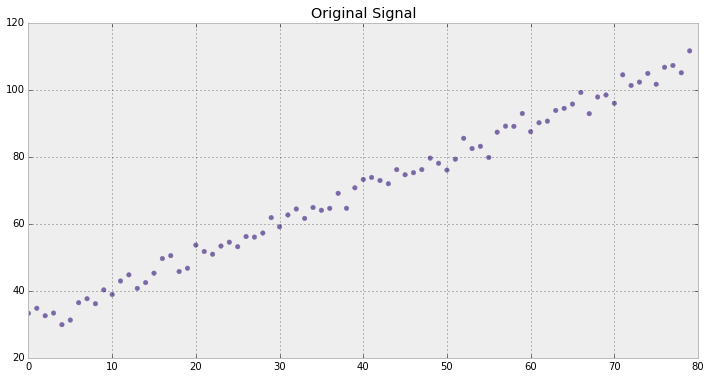In :
plt.figure(figsize=(12, 6));
plt.scatter(range(y_with_outlier.size), y_with_outlier, color="#7A68A6");
plt.title('Signal with Outliers');
plt.xlim([0, len(y_with_outlier)]);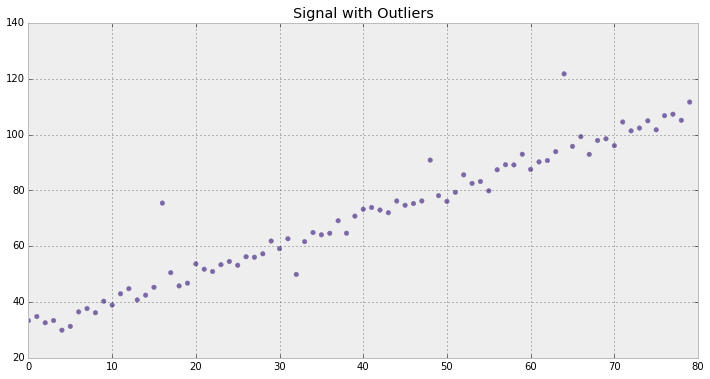Outliers are much more distant than the noise so visually it is easy to detect in general. In this application, I choose a basic linear signal, but as long as the signal can be modeled(however all of the models are wrong), then I could capture outliers(do not forget some models are useful).

## Priors¶

All of the priors are chosen as uniform as I do not assume any prior knowledge where the outliers may occur in the signal. If you have prior knowledge of what might be outliers look like in the distribution, this would help both the inference as well as the outlier detection. If you do not have the priors, the data will lead the way eventually.

If the component that measures the signal has a failure rate, that probability could be easily incorporated to our model. Especially, if you have small data(nowadays, everyone has big, but just for the sake argument), those priors would become handy in order to learn the posterior distribution of the variables that you are interested.

$$p_{outlier} = U(0, 1)$$$$p_{mean-outlier} = U(-100, 100)$$$$p_{spread-outlier} = U(-100, 100)$$
In :
outlier_points = pymc.Uniform('outlier_points', 0, 1.0, value=0.1)
mean_outliers = pymc.Uniform('mean_outliers', -100, 100, value=0)


## Models¶

I define basic linear regression as model and sample slope and intercept assuming the signal that shows linearity results in higher posterior probabilities than the ones that do not obey(read outliers). The inlier points are chosen from Bernoulli distribution: $$p_{inlier} = B(n, 1-p_{outlier})$$ where $n$ is the signal length. Note that I also define another posterior computation for outlier based on gaussian distribution in this section. This will be used to model the outliers in the next cell. The idea behind this approach, the distant observations(some call outliers), will have lower posterior, and by doing so I could identify them.

In :
@pymc.stochastic
def slope_and_intercept(value=[1., 10.]):
slope, intercept = value
prob_slope = np.log(1. / (1. + slope ** 2))
return prob_slope

@pymc.deterministic
def model_(x=x, slope_and_intercept=slope_and_intercept):
slope, intercept = slope_and_intercept
fit = slope * x + intercept
return fit

inlier = pymc.Bernoulli('inlier', p=1 - outlier_points, value=np.zeros(x.size))

inlier_posterior = np.sum(inlier * (np.log(2 * np.pi * spread ** 2) + (y_with_outlier - mu) ** 2 / (spread ** 2)))
outlier_posterior = np.sum((1 - inlier) * (np.log(2 * np.pi * ((spread ** 2) + (spread_outliers ** 2))) + (y_with_outlier - mean_outliers) ** 2 / ((spread ** 2) + (spread_outliers ** 2))))
return -0.5 * (inlier_posterior + outlier_posterior)


If you want to define your own distribution(or wrap some distribution for your own purposes), PyMC supports both stochastic_from_dist and stochastic_from_data to get your custom distributions. Then, you wrap all of the models and variables into one model to infer the unknowns. I am interested in the outliers so I define our outlier model based on Gaussian model where I would use inlier and outlier mean and spread to capture the differences between inlier and outlier distribution. The basic assumption is that the spread of the outlier as you could see from the graph is larger than inliers. This stems from the definition of the outlier as it could be defined as the the most distant observations from the distribution. I will use the posterior likelihood of the outlier in the custom distribution.(mv just says the variable is in the array form, what it stands for is beyond my faculty, though.

In :
outlier_distribution = pymc.stochastic_from_dist('outlier_distribution',
logp=log_posterior_likelihood_of_outlier,
dtype=np.float,
mv=True)

outlier_dist = outlier_distribution('outlier_dist',
mu=model_,
mean_outliers=mean_outliers,
inlier=inlier,
observed=True,
value=y_with_outlier)

model = dict(outlier_dist=outlier_dist,
slope_and_intercept=slope_and_intercept,
model_=model_,
inlier=inlier,
outlier_points=outlier_points,
mean_outliers=mean_outliers,
)


## Inference¶

After getting the posterior probabilities, I will get the outliers based on the probability. The points that have smaller probabilities than some threshold become our outliers. If I want to be more restrictive on outliers, I could put a smaller threshold. Then, I would get only very distant outliers, whereas by increasing the probability I would capture the signal which has low variance. Threshold in here exists but this threshold is actually a probability measure of the posterior distribution, so it is very useful. Since it is not arbitrary, it generalizes better than the thresholds that are put in the frequentist approaches.

In :
prob_threshold = 0.40

mcmc = pymc.MCMC(model)
mcmc.sample(100000, 20000)

slope_and_intercept_trace = mcmc.trace('slope_and_intercept')[:]
model_trace = mcmc.trace('model_')[:]
outlier_points_trace = mcmc.trace('outlier_points')[:]

probability_of_points = mcmc.trace('inlier')[:].astype(float).mean(0)
outlier_x = x[probability_of_points < prob_threshold]
outlier_y = y_with_outlier[probability_of_points < prob_threshold]

 [-----------------100%-----------------] 100000 of 100000 complete in 143.8 sec

## Posterior Plots¶

In :
fix, ax = plt.subplots(figsize=(16,16))

ax = plt.subplot(411)
plt.hist(slope_and_intercept_trace[:,0], histtype='stepfilled', bins=30, alpha=.7,
color="#A60628", normed=True);
plt.legend(loc="upper left");
plt.title("Posterior distributions");
plt.ylim([0, 50]);
plt.ylabel("Slope");

ax = plt.subplot(412);
plt.hist(slope_and_intercept_trace[:,1], histtype='stepfilled', bins=30,
alpha=.7, color="#7A68A6", normed=True);
plt.legend(loc="upper left");
plt.ylim([0, 2]);
plt.ylabel("Intercept");

plt.subplot(413);
plt.hist(outlier_points_trace, histtype='stepfilled', bins=100, alpha=.7,
color="#348ABD",  normed=True);
plt.ylabel("Outlier Point Probability in Signal");

plt.subplot(414);
color="#188487",  normed=True);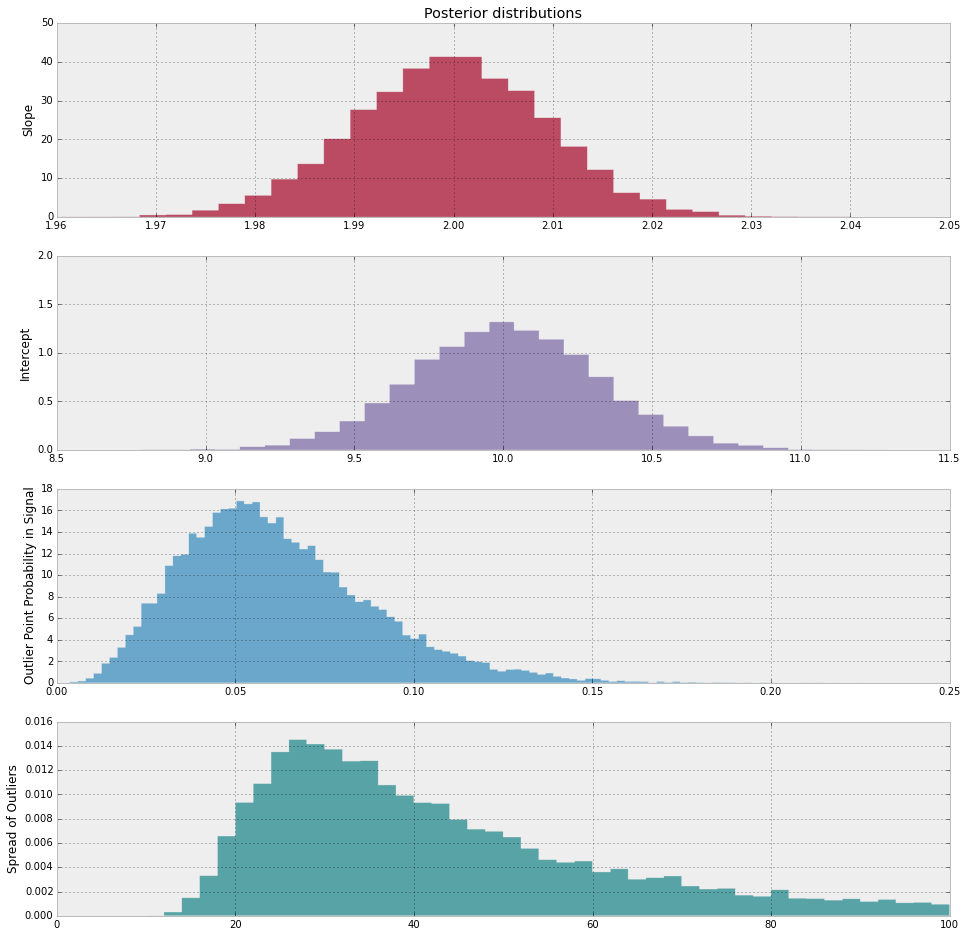The posterior is good in terms of, I remove most of our uncertainties(they do not look like uniform). Slope is max around 2, intercept gets maximized around 10, which are all good and very close to the main signal values. What else, I could observe spread of the outliers and what could be the ratio of outliers in the total signal, which are also good. I could also get estimate probabilities of spread in outliers and ratio of outliers in the signal which are very important measures in general even if they are not directly used for outlier detection.

## Outlier Plots¶

In :
fig = plt.figure(figsize=(12, 12));
plt.boxplot([y_with_outlier, y]);
plt.xticks([1, 2], ['Outlier Signal', 'Original Signal']);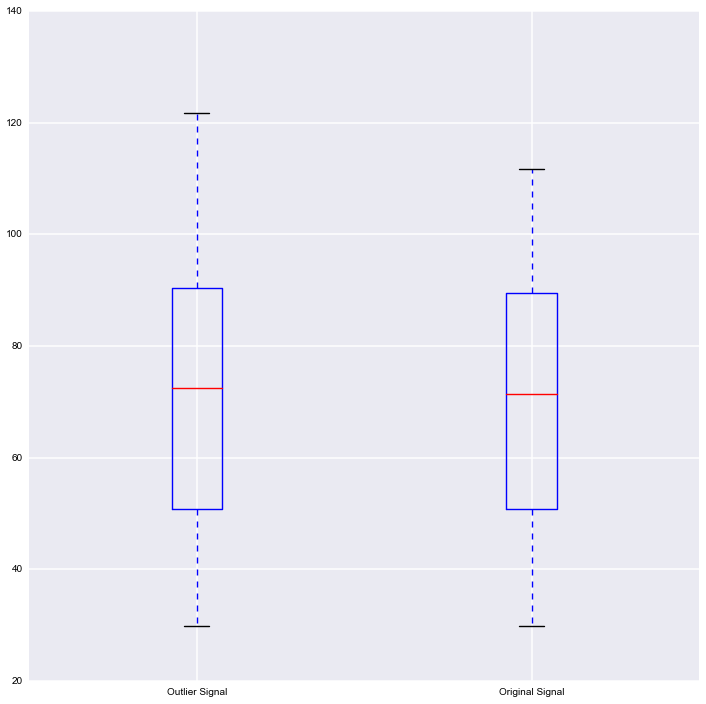As expected, the outlier signal spread is larger than the original signal due to outliers, which could be quick sanity check for our methods before plotting the original signal along with outliers. Note that the mean change for different signals, especially the signals that vary a lot in time does not really show anything. I am trying to minimize the spread(variance) of the signal rather than mean. It just happens to be the outlier signal values have higher signal amplitude than the original signal.

In :
fig, ax = plt.subplots(figsize=(12, 8))
ax.scatter(x, y_with_outlier, c='#7A68A6', lw=1, label='Original Signal')
ax.scatter(outlier_x, outlier_y,  facecolors='#A60628', edgecolors='#7A68A6', label='Outliers', lw=1, s=100, alpha=0.2)

ax.set_xlim(np.min(x)-10, np.max(x) + 30);
ax.set_ylim(np.min(y_with_outlier)-10, np.max(y_with_outlier) + 20);
ax.set_title('Original Signal and Outliers');
plt.legend();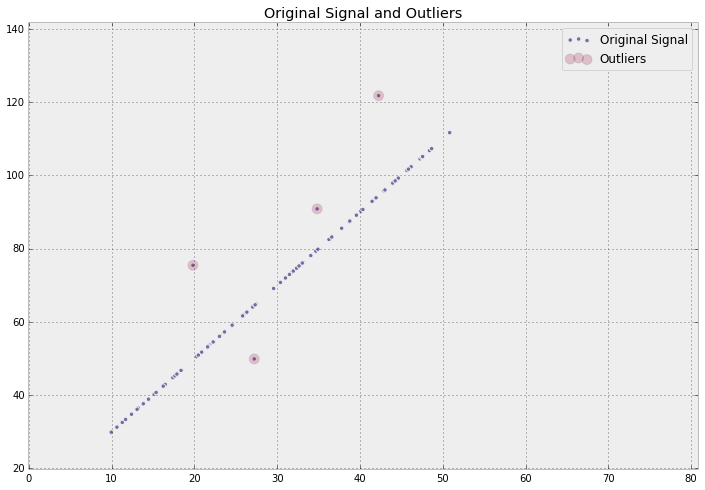Outliers are nicely captured in the signal. Note that since the scale is somehow large, the signal seems to not having any noise, but it is not perfectly linear.

I do not want to go into the discussion of Bayesian is better than Frequentist, but here are my observations for this specific case of advantages and disadvantages of Bayesian approach cin comparison with Frequentist.

• Unlike Median Filtering and FFT, which requires a lot of parameters, MCMC requires only probability threshold.
• As long as the model is nicely captured, the priors do not have to be known beforehand. The data leads the way.
• If priors are known, they could be incorporated into the model whereas other models cannot use prior information.
• Probabilistic, you could reason about probabilities whereas frequentist approaches do not provide a similar functionality like Bayesain methods.
• We could build complex models, consider the outliers in this example. In Frequentist approaches, there is no similar mechanism to build such a system.